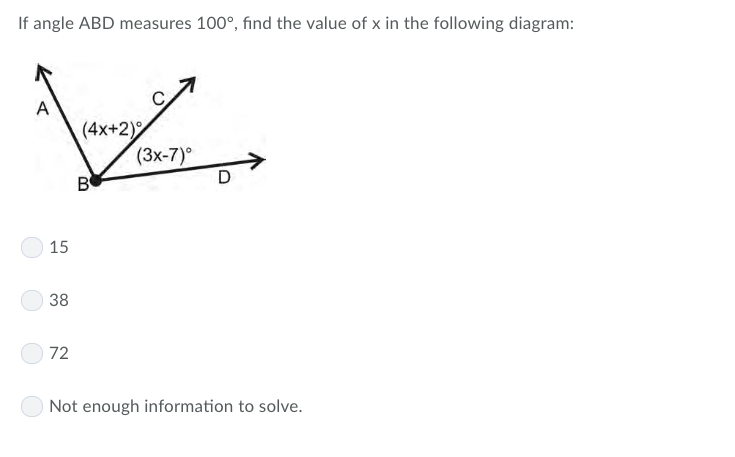# If angle ABD measures 100°, find the value of x in the following diagram:(4x+2)(3x-7)°153872Not enough information to solve

Question
828 viewshelp_outlineImage TranscriptioncloseIf angle ABD measures 100°, find the value of x in the following diagram: (4x+2) (3x-7)° 15 38 72 Not enough information to solve fullscreen
check_circle

Step 1

Here given that angle ABD is 1000. as shown in figure.

Angle ABC = (4x+2)0   Angle CBD = (3x-7)0

Step 2

We know that Angle ABD is the sum of angle ABC and angle CBD. So we can write

Angl...

### Want to see the full answer?

See Solution

#### Want to see this answer and more?

Solutions are written by subject experts who are available 24/7. Questions are typically answered within 1 hour.*

See Solution
*Response times may vary by subject and question.
Tagged in

### Other